# Semi-Parametric Neural Image Synthesis

Andreas Blattmann*, Robin Rombach*, Kaan Oktay, Jonas Müller, Björn Ommer

LMU Munich, MCML & IWR, Heidelberg University, Germany

* equal contribution

## Abstract

Novel architectures have recently improved generative image synthesis leading to excellent visual quality in various tasks. Much of this success is due to the scalability of these architectures and hence caused by a dramatic increase in model complexity and in the computational resources invested in training these models. Our work questions the underlying paradigm of compressing large training data into ever growing parametric representations. We rather present an orthogonal, semi-parametric approach. We complement comparably small diffusion or autoregressive models with a separate image database and a retrieval strategy. During training we retrieve a set of nearest neighbors from this external database for each training instance and condition the generative model on these informative samples. While the retrieval approach is providing the (local) content, the model is focusing on learning the composition of scenes based on this content. As demonstrated by our experiments, simply swapping the database for one with different contents transfers a trained model post-hoc to a novel domain. The evaluation shows competitive performance on tasks which the generative model has not been trained on, such as class-conditional synthesis, zero-shot stylization or text-to-image synthesis without requiring paired text-image data. With negligible memory and computational overhead for the external database and retrieval we can significantly reduce the parameter count of the generative model and still outperform the state-of-the-art.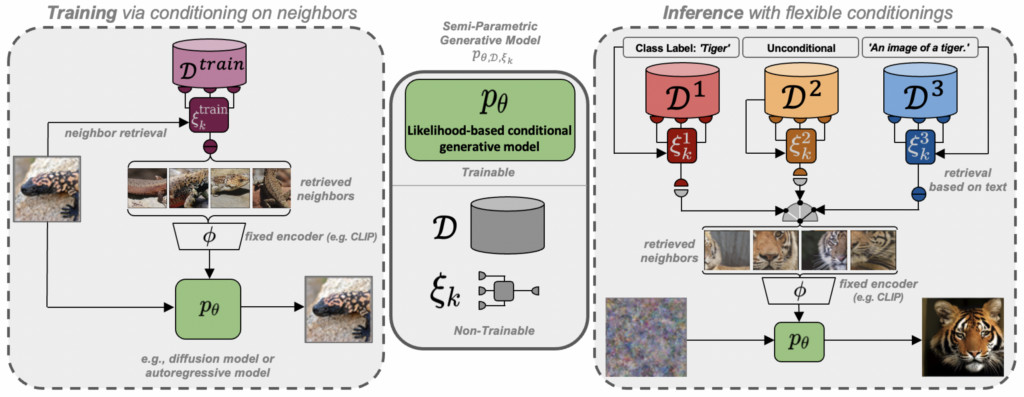Figure 3: A semi-parametric generative model consists of a trainable conditional generative model (decoding head) $$p_\theta(x \vert \cdot)$$, an external database $$\mathcal{D}$$ containing visual examples and a sampling strategy $$\xi_k$$ to obtain a subset $$\mathcal{M}_{\mathcal{D}}^{(k)} \subseteq \mathcal{D}$$, which serves as conditioning for $$p_\theta$$. During training, $$\xi_k$$ retrieves the nearest neighbors of each target example from $$\mathcal{D}$$, such that $$p_\theta$$ only needs to learn to compose consistent scenes based on $$\mathcal{M}_{\mathcal{D}}^{(k)}$$, see Sec. 3.2. During inference, we can exchange $$\mathcal{D}$$ and $$\xi_k$$, thus resulting in flexible sampling capabilities such as post-hoc conditioning on class labels ($$\xi_k^1$$) or text prompts ($$\xi_k^3$$), cf Sec. 3.3, and zero-shot stylization, cf Sec. 4.3.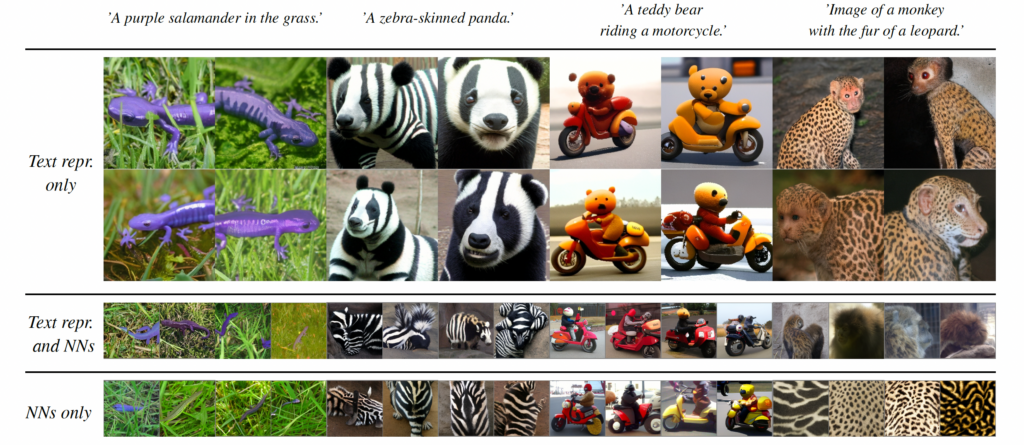Figure 2: As we retrieve nearest neighbors in the shared text-image space provided by CLIP, we can use text prompts as queries for exemplar-based synthesis. We observe our RDM to readily generalize to unseen and fictional text prompts when building the set of retrieved neighbors by directly conditioning on the CLIP text encoding $$\phi_{\text{CLIP}}(c_{\text{text}})$$ (top row). When using $$\phi_{\text{CLIP}}(c_{\text{text}})$$ together with its $$k-1$$ nearest neighbors from the retrieval database (middle row) or the $$k$$ nearest neighbors alone without the text representation, the model does not show these generalization capabilities (bottom row).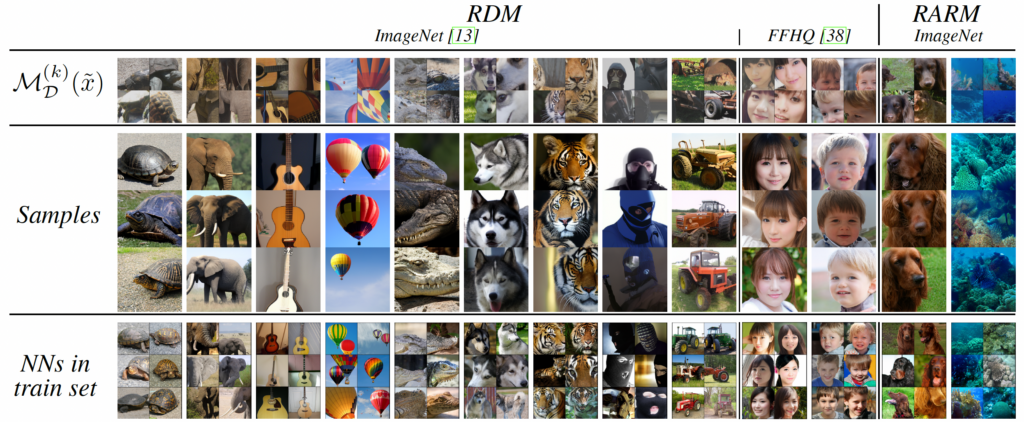Figure 5: Samples from our unconditional models together with the sets of $$\mathcal{M}_{\mathcal{D}}^{(k)}(\tilde{x})$$ of retrieved neighbors for the pseudo query $$\tilde{x}$$, cf. Sec. 3.3, and nearest neighbors from the train set, measured in CLIP  feature space. For ImageNet samples are generated with $$m=0.01$$, guidance with $$s = 2.0$$ and 100 DDIM steps for RDM and $$m=0.05$$, guidance scale $$s=3.0$$ and top-$$k=2048$$ for RARM. On FFHQ we use $$s=1.0$$, $$m=0.1$$.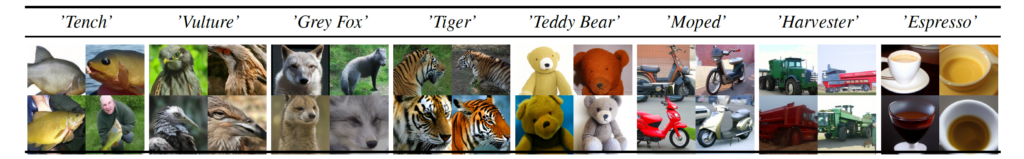Figure 10: RDM can be used for class-conditional generation on ImageNet despite being trained without class labels. To achieve this during inference, we compute a pool of nearby visual instances from the database $$\mathcal{D}$$ for each class label based on its textual description, and combine it with its $$k-1$$ nearest neighbors as conditioning.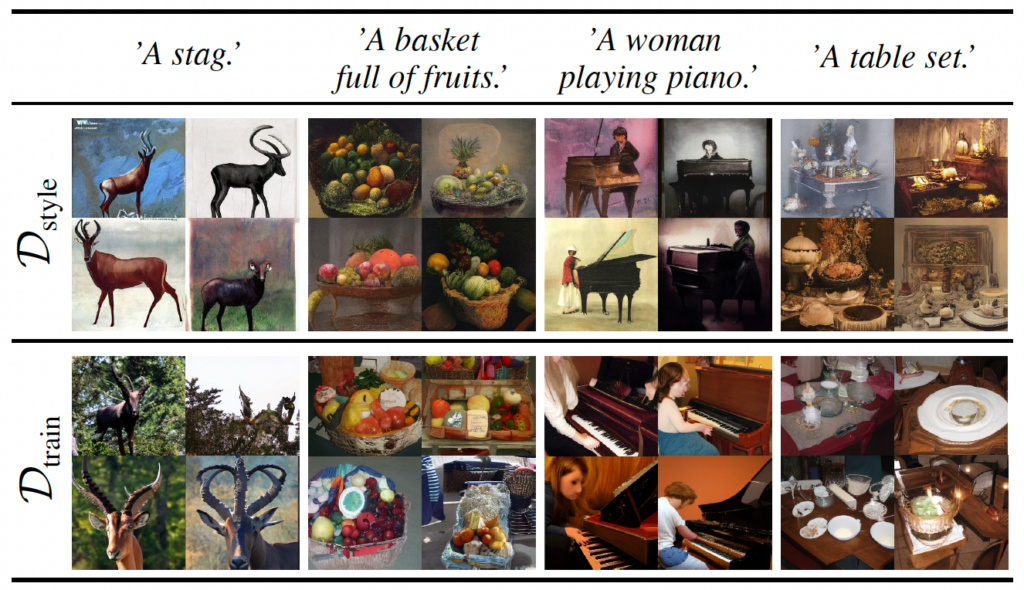Figure 11: Zero-shot text-guided stylization with our ImageNet-RDM.